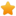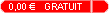565 tutoriels disponibles
Chercher un tutoriel
Bienvenue
sur ShareannonceEcrit par: Shareannonce
Date création:  09-09-2018
Nombre de vues:  1463
Catégorie:  crypto-monnaie
Note:Tutoriel N° 2bc

Why was 21 million of bitcoins created?

Here's a mathematical explanation:

Calculate the number of blocks per 4 year cycle:

6 blocks per hour
* 24 hours per day
* 365 days per year
* 4 years per cycle
= 210,240
~= 210,000

Sum all the block reward sizes:

50 + 25 + 12.5 + 6.25 + 3.125 + ... = 100

Multiply the two:

210,000 * 100 = 21 million

Economically, because the currency is effectively infinitely divisible, then the precise amount doesn't matter, as long as the limit remains fixed.

Autres tutoriels de la même catégorie >

ShareAnnonce version 2.0 Tous droits reserves. | Condition d'utilisation | Contact